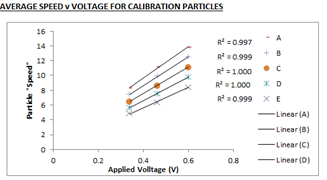# What are the performance indicators for good zeta potential measurements?

Assess particle speed vs voltage relationship

Average particle speed and applied voltage have a linear relationship, with the speed increasing with rising voltage. The R^2 value should be typically larger than 0.98. If the particle speed does not change or even decreases with increasing applied voltage, please select another pore for the zeta potential measurement.Assess the zeta potential variance

The zeta potential variance is a measure of how accurately an individual blockade has been “mapped” relative to the calibration particle recordings.  It is not a statistical measurement of the whole population, and relates only to the calculated zeta potential value for each particle and not the particle diameter.

The zeta potential of each particle is calculated at five temporal points as it passes through the nanopore.  The mean zeta potential is plotted, and a variance is allocated to that measurement.

If a particle zeta potential measurement has been taken, using poor calibration data, incorrect settings or with high background noise, the variance of that measurement is likely to be high.  By graduating the size and transparency of points on the scatter plot, the user can see where the higher quality (lower variance) data is.  The limits are applied to all plotted data sets.In the examples above, the variance limits have been adjusted from 10 and 20 mV (left) to 0.02 and 0.5 mV (right).  The sample measurements have low variances, and are an example of excellent data. Please note, that no data points are excluded due to high variance. All  data points are always plotted.